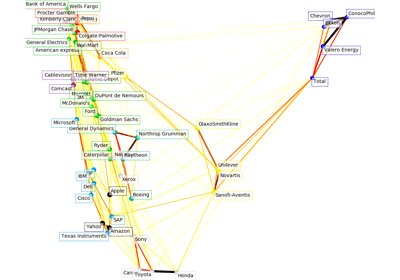/scikit-learn

# sklearn.cluster.affinity_propagation

`sklearn.cluster.affinity_propagation(S, preference=None, convergence_iter=15, max_iter=200, damping=0.5, copy=True, verbose=False, return_n_iter=False)` [source]

Perform Affinity Propagation Clustering of data

Read more in the User Guide.

Parameters: `S : array-like, shape (n_samples, n_samples)` Matrix of similarities between points `preference : array-like, shape (n_samples,) or float, optional` Preferences for each point - points with larger values of preferences are more likely to be chosen as exemplars. The number of exemplars, i.e. of clusters, is influenced by the input preferences value. If the preferences are not passed as arguments, they will be set to the median of the input similarities (resulting in a moderate number of clusters). For a smaller amount of clusters, this can be set to the minimum value of the similarities. `convergence_iter : int, optional, default: 15` Number of iterations with no change in the number of estimated clusters that stops the convergence. `max_iter : int, optional, default: 200` Maximum number of iterations `damping : float, optional, default: 0.5` Damping factor between 0.5 and 1. `copy : boolean, optional, default: True` If copy is False, the affinity matrix is modified inplace by the algorithm, for memory efficiency `verbose : boolean, optional, default: False` The verbosity level `return_n_iter : bool, default False` Whether or not to return the number of iterations. `cluster_centers_indices : array, shape (n_clusters,)` index of clusters centers `labels : array, shape (n_samples,)` cluster labels for each point `n_iter : int` number of iterations run. Returned only if `return_n_iter` is set to True.

#### Notes

For an example, see examples/cluster/plot_affinity_propagation.py.

When the algorithm does not converge, it returns an empty array as `cluster_center_indices` and `-1` as label for each training sample.

When all training samples have equal similarities and equal preferences, the assignment of cluster centers and labels depends on the preference. If the preference is smaller than the similarities, a single cluster center and label `0` for every sample will be returned. Otherwise, every training sample becomes its own cluster center and is assigned a unique label.

#### References

Brendan J. Frey and Delbert Dueck, “Clustering by Passing Messages Between Data Points”, Science Feb. 2007

## Examples using `sklearn.cluster.affinity_propagation`Visualizing the stock market structure